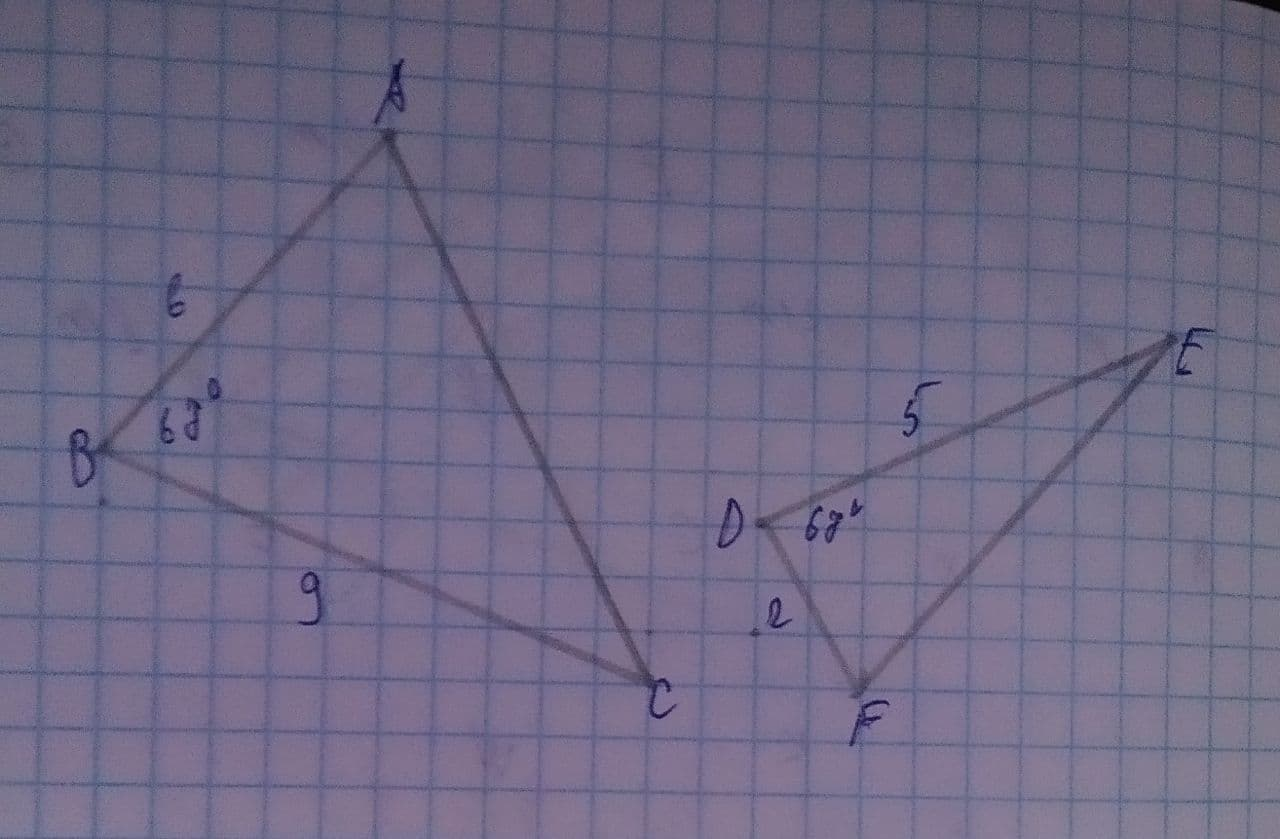# Can the triangles below be proven similar by the SAS Similarity Postulate?midtlinjeg 2021-07-31 Answered
Can the triangles below be proven similar by the SAS Similarity Postulate?Yes, the SAS Similarity Postulate can be used because 2 sets of corresponding sides are proportional, and the included angles by these sides are congruent.
Yes, the SAS Similarity Postulate can be used because 2 sets of corresponding sides are congruent, and the included angles by these sides are congruent.
No, the SAS Similarity Postulate cannot be used because the corresponding angles are congruent but not included by the proportional sides.
No, the SAS Similarity Postulate cannot be used because the 2 sets of corresponding sides are not proportional.
You can still ask an expert for help

• Questions are typically answered in as fast as 30 minutes

Solve your problem for the price of one coffee

• Math expert for every subject
• Pay only if we can solve itStrycharzT

Step 1
here we have triangles and if we use the SAS similarity postulate we have to make sure to use any two sides and one angle from each triangle respectively such as

or

Step 2
using either of any two ratios we get

but $1.2\ne 4.5$
or

but $3\ne 1.8$
Step 3
so, we can see that neither of them is satisfying the SAS rule as their corresponding angles are equal but their corresponding sides are not proportional.
therefore, the correct option is D